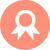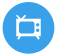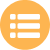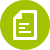## 二建-建筑工程## 监理案例分析# 安徽省司索信号工在线测试模拟试题

A.吊钩下降

B.吊钩水平移动

C.吊钩微微上升

D.吊钩上升

A.10.3mm

B.9.0mm

C.9.3mm

D.9.5mm

A.载荷距高

B.运动距离

C.安全距离

D.运行距离

A.吊钩下降

B.吊钩水平移动

C.要副钩

D.吊钩上升

A.每次使用过程

B.每次使用前

C.每次使用后

D.每次使用中

A.转臂

B.微微伸臂

C.升臂

D.降臂

A.起重伤害

B.物体打击

C.高处坠落

D.机械伤害

A.通用手势

B.专用手势

C.特殊手势

D.专用音响

A.时松时紧

B.时刻绷紧

C.时刻放松

D.瞬间松弛

A.严禁

B.可以

C.不得

D.允许

A.立即更换

B.立即报废

C.立即报停

D.减载使用

A.冲顶滞留

B.冲顶失速

C.冲顶坠落

D.冲顶下滑

A.紧急停止

B.工作结束

C.指示降落方位

D.停止运行

A.禁止

B.允许

C.可以

D.不得

A.防倾倒措施

B.防风措施

C.加固措施

D.卸载措施

A.40°-60°

B.30°-60°

C.45°-60°

D.45°-70°

A.最大高度

B.最小距离

C.最大幅度

D.最小幅度

A.顺风方向

B.逆风方向

C.上风方向

D.下风方向

A.物体变态

B.物体释放

C.物体运动

D.物体转移

A.时松时紧

B.始终松弛

C.始终紧张

D.可松可紧

A.密度

B.质量

C.重量

D.重物

A.最大高度

B.最小距离

C.最大幅度

D.最小幅度

A.各二次

B.各一次

C.各三次

D.各四次

A.吊钩水平微微移动

B.吊钩水平移动

C.吊钩微微上升

D.吊钩微微下落

A.起升高度

B.起升幅度

C.变幅高度

D.起升速度

A.金属机构

B.金属结构

C.金属套管

D.金属护套

A.4m

B.3m

C.2m

D.5m

A.尽量快进

B.尽量慢速

C.尽量一致

D.尽量异步

A.70%

B.75%

C.80%

D.85%

A.小于8倍

B.大于6倍

C.小于6倍

D.小子5倍

A.80%

B.100%

C.90%

D.95%

A.吊钩水平微微移动

B.微动范围

C.指示降落方位

D.吊钩微微下落

A.吊钩下降

B.要主钩

C.要副钩

D.吊钩上升

A.15倍

B.10倍

C.20倍

D.30倍

A.无滑扣

B.无锈蚀

C.无损伤

D.无腐蚀

A.严禁有人

B.严禁站人

C.可以站人

D.不得站人

A.绑扎牢靠

B.放置稳定

C.有人扶着

D.可靠固定

A.微速起钩

B.慢速落钩

C.慢速起钩

D.微速落钩

A.高处坠落

B.触电伤害

C.起重伤害

D.机械伤害

A.550mm

B.400mm

C.500mm

D.450mm

A.预备

B.要主钩

C.要副钩

D.吊钩上升

A.起重条件

B.稳定条件

C.吊物条件

D.平衡条件

A.非违章操作

B.行为过失

C.行为不当

D.违规行为

A.转臂

B.微微伸臂

C.微微降臂

D.降臂

A.起重臂下

B.作业半径

C.起重旋转半径

D.吊装区域

A.滑移法

B.平移法

C.旋转法

D.递送法

A.预备

B.要主钩

C.起吊

D.要副钩

A.720°

B.540

C.280°

D.360°

A.起重量

B.额定起重量

C.起重力矩

D.最大起重量

A.起重机械

B.运输机械

C.吊装设备

D.卷扬机械

A.避开吊载

B.避开吊车

C.避开人员

D.避开障碍物

E.加速提升

A.牢固.严密

B.完整有效

C.易于拆卸

D.不易拆卸

E.无法拆卸

A.搁置点

B.拉结点

C.支撑点

D.锚固点

E.附加点

A.左手指挥臂杆

B.右手指挥臂杆

C.右手指挥吊钩

D.左手指挥吊钩

E.双手在胸前交叉

A.支承面越小

B.支承面越大

C.重心越高

D.重心越低

E.附加点少

A.钳爪与物品开口度

B.摩擦功能

C.钳爪与物品接触面积

D.摩擦系数

E.导热性

A.要主钩

B.要副钩

C.微动范围

D.手势或旗语时

E.休息

A.手拳

B.手指

C.手臂

D.手心

E.臂部

A.合成纤维吊装带

B.钢丝绳吊索

C.吊链

D.其他连接件

E.安全锁

A.0.5t及以上升降机

B.40t.m及以上塔式起重机

C.300t/h及以上装卸桥

D.机械式停车设备

E.物料装卸机

A.手心

B.手指

C.手臂

D.握拳

E.腰部

A.整根绳索制成

B.中间不得有接头

C.中间可以有接头

D.环形吊索只允许有以处接头

E.环形吊索只允许有两处接头

A.单机吊装

B.双机抬吊

C.三机抬吊

D.相互抬吊

E.多机抬吊

A.吊钩

B.臂杆

C.机械位移

D.吊物

E.吊绳

A.平头式塔式起重机

B.锤头或尖头式塔式起重机

C.折臂变幅塔式起重机

D.矩形塔式起重机

E.小车变幅塔式起重机

A.建筑起重机

B.流动式起重机

C.矿井提升设备

D.载人电梯设备

E.物料提升机

A.吊钩完好

B.链条完整

C.齿轮啮合不良

D.自锁装置

E.绑扎牢固

A.轮槽

B.轮轴

C.夹板

D.吊钩

E.防护罩

A.变速齿轮沿轴转动

B.啮合正确

C.无杂音

D.润滑良好

E.干净整洁

A.插接

B.打结

C.绳扣

D.螺栓

E.绳卡

A.正确

B.错误

A.正确

B.错误

A.正确

B.错误

A.正确

B.错误

A.正确

B.错误

A.正确

B.错误

A.正确

B.错误

A.正确

B.错误

A.正确

B.错误

A.正确

B.错误

A.正确

B.错误

A.正确

B.错误

A.正确

B.错误

A.正确

B.错误

A.正确

B.错误

A.正确

B.错误

A.正确

B.错误

A.正确

B.错误

A.正确

B.错误

A.正确

B.错误

##### 版权声明

 留言与评论（共有 0 条评论）

ock123.com

### 蜀ICP备18034711号.